# ML Aggarwal Class 6 Solutions for ICSE Maths Chapter 2 Whole Numbers Check Your Progress

## ML Aggarwal Class 6 Solutions for ICSE Maths Chapter 2 Whole Numbers Check Your Progress

Question 1.
Write next three consecutive whole numbers of the number 9998.
Solution: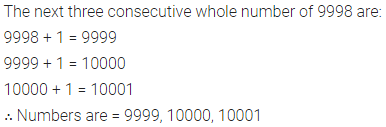Question 2.
Write three consecutive whole numbers occurring just before 567890.
Solution:Question 3.
Find the product of the successor and the predecessor of the smallest number of 3-digits.
Solution: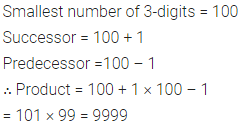Question 4.
Find the number of whole numbers between the smallest and the greatest numbers of 2-digits.
Solution:Question 5.
Find the following sum by suitable arrangements:
(i) 678 + 1319 + 322 + 5681
(ii) 777 + 546 + 1463 + 223 + 537
Solution: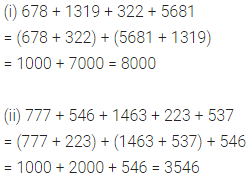Question 6.
Determine the following products by suitable arrangements:
(i) 625 × 437 × 16
(ii) 309 × 25 × 7 × 8
Solution: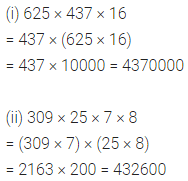Question 7.
Find the value of the following by using suitable properties:
(i) 236 × 414 + 236 × 563 + 236 × 23
(ii) 370 × 1587 – 37 × 10 × 587
Solution: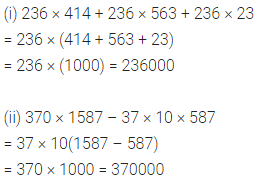Question 8.
Divide 6528 by 29 and check the result by division algorithm.
Solution: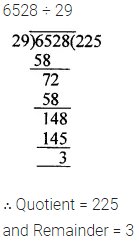Question 9.
Find the greatest 4-digit number which is exactly divisible by 357.
Solution: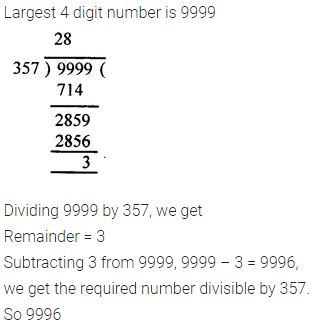Question 10.
Find the smallest 5-digit number which is exactly divisible by 279.
Solution: